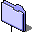Groups & Graphs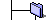G&G Home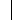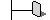G&G MacintoshG&G WindowsNewsContact InfoAlgorithmsLinks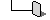Requests/Bug ReportsUsing G&GGraph/Digraph OverviewGroup OverviewTorus Map OverviewHexagon Map OverviewProjective Map OverviewSphere Map OverviewProjective Configurations OverviewPolyhedron Overview4D Polytope OverviewFractal Overviewn-Body System OverviewDownloadsG&G Binaries (ftp)G&G Graph LibraryUploadsGraph LibraryQ4, the 4-cubeK3,3 embeddings on the torusK5 embeddings on the torusK6 embeddings on the torusThe embeddings of the cube on the torusK5 and K6 embeddings on the projective planeK3,3 and K3,4 embeddings on the projective plane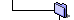Vertex-transitive graphs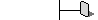Vertex-transitive graphs on 8 verticesVertex-transitive graphs on 9 verticesVertex-transitive graphs on 10 verticesVertex-transitive graphs on 11 verticesVertex-transitive graphs on 12 vertices, 18 edgesVertex-transitive graphs on 12 vertices, 24 edgesVertex-transitive graphs on 12 vertices, 30 edgesVertex-transitive graphs on 13 verticesVertex-transitive graphs on 14 vertices, 21 and 28 edgesVertex-transitive graphs on 14 vertices, 35 edgesVertex-transitive graphs on 14 vertices, 42 edgesVertex-transitive graphs on 15 vertices, 30 edges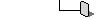Vertex-transitive graphs on 15 vertices, 45 edgesSite Index: Easily find your way around the Groups & Graphs website